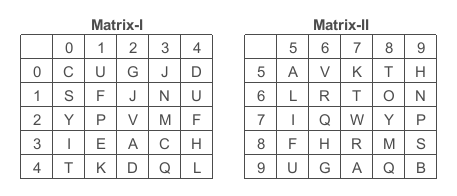Question 3

# A word is represented by one set of numbers as given in any one of the alternatives. The sets of numbers given in the alternatives are represented by two classes of alphabets as shown in the given two matrices. The column and rows of Matrix-I are numbered from 0 to 4 and that of Matrix-II are numbered from 5 to 9. A letter these matrices can be represented first by its columns, for example, 'U' can be represented by 14, 95, etc., and 'M' can be represented by 23, 88, etc, similarly, you have to identify set for the word "PARK".Solution

(A) : 21, 55, 67, 41 = PATK

(B) : 79, 55, 87, 41 = PARK

(C) : 21, 31, 67, 57 = PETK

(D) : 79, 32, 67, 57 = PATK

=> Ans - (B)